Next: Magnetic monopoles? Up: Time-independent Maxwell equations Previous: The Lorentz force

## Ampère's law

Magnetic fields, like electric fields, are completely superposable. So, if a fieldis generated by a current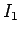flowing through some circuit, and a fieldis generated by a current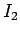flowing through another circuit, then when the currentsandflow through both circuits simultaneously the generated magnetic field is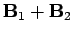.Consider two parallel wires separated by a perpendicular distanceand carrying electric currentsand, respectively (see Fig. 30). The magnetic field strength at the second wire due to the current flowing in the first wire is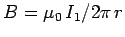. This field is orientated at right-angles to the second wire, so the force per unit length exerted on the second wire is(253)

This follows from Eq. (229), which is valid for continuous wires as well as short test wires. The force acting on the second wire is directed radially inwards towards the first wire. The magnetic field strength at the first wire due to the current flowing in the second wire is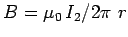. This field is orientated at right-angles to the first wire, so the force per unit length acting on the first wire is equal and opposite to that acting on the second wire, according to Eq. (229). Equation (253) is sometimes called Ampère's law, and is clearly another example of an action at a distance law: i.e., if the current in the first wire is suddenly changed then the force on the second wire immediately adjusts. In reality, there should be a short time delay, at least as long as the propagation time for a light signal between the two wires. Clearly, Ampère's law is not strictly correct. However, as long as we restrict our investigations to steady currents it is perfectly adequate.Next: Magnetic monopoles? Up: Time-independent Maxwell equations Previous: The Lorentz force
Richard Fitzpatrick 2006-02-02